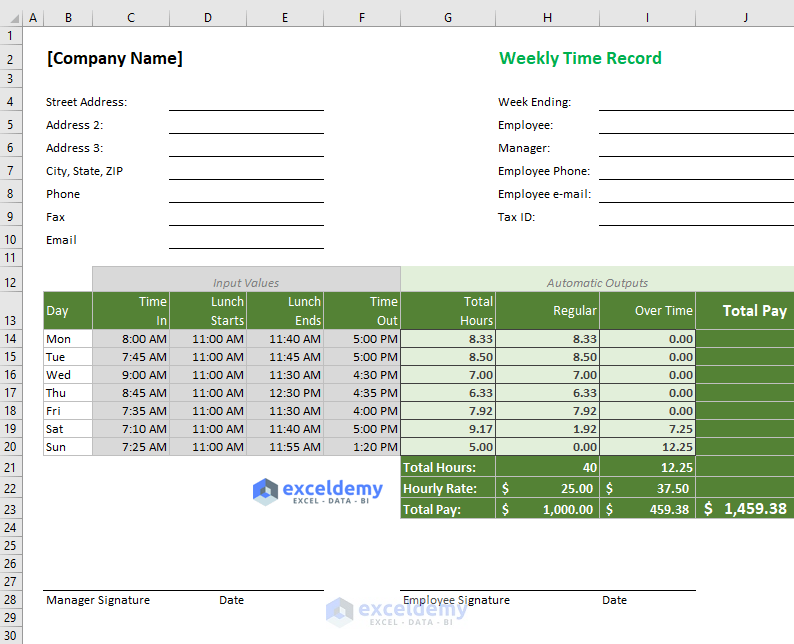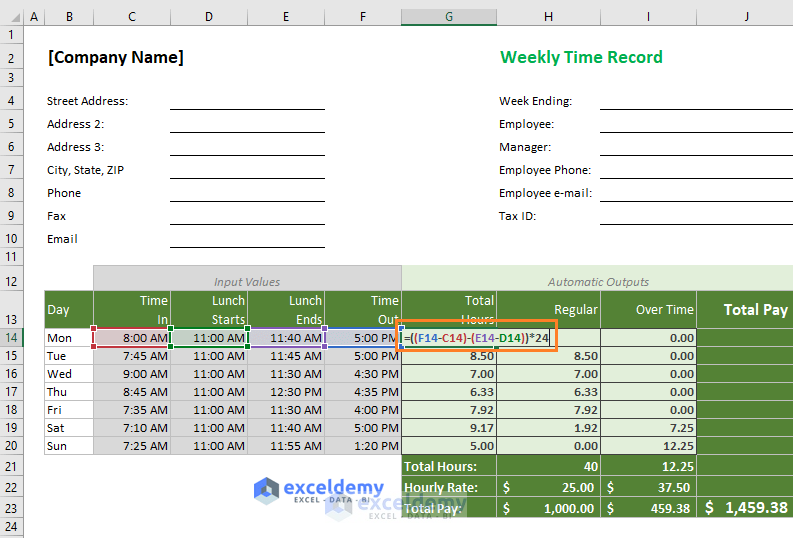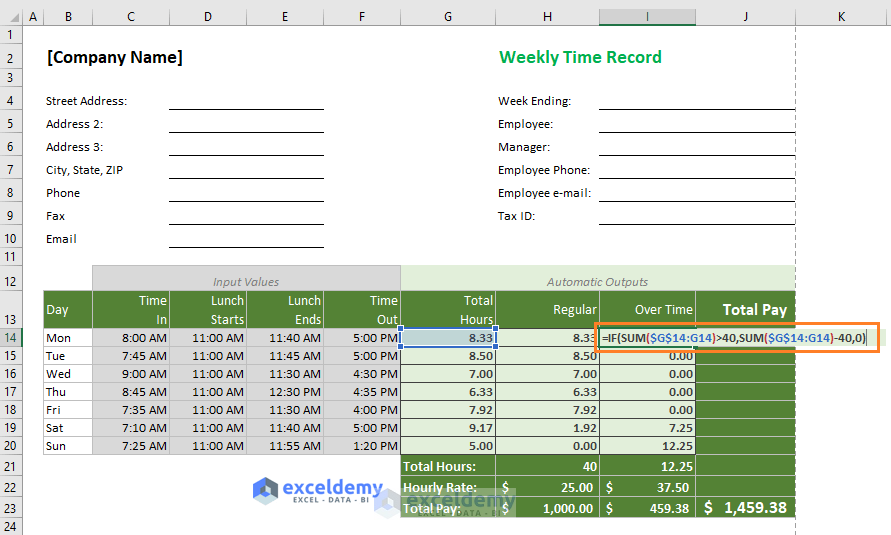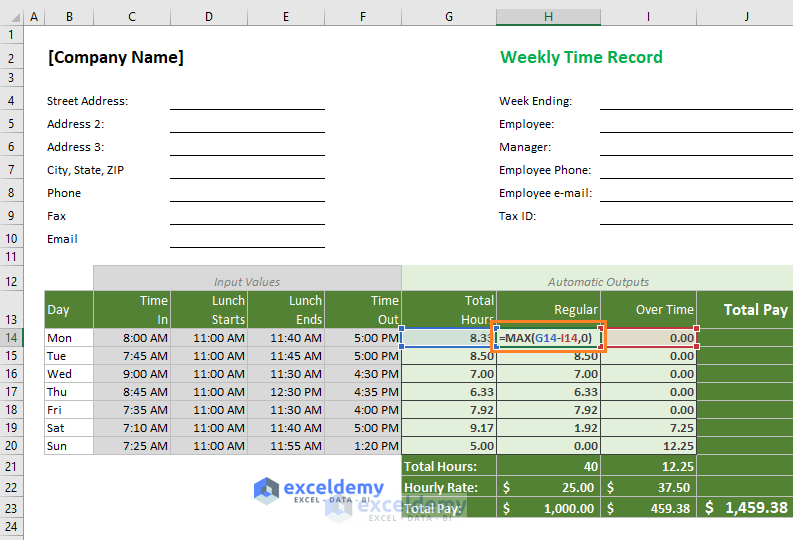Disclosure: This post may contain affiliate links, meaning when you click the links and make a purchase, we receive a commission.

# Excel Formula for Overtime over 40 Hours [with Free Template]In this article, you will get the Excel formula for calculating overtime over 40 hours. The Excel template is ready for your daily use.

Different companies calculate overtime in different ways. But the most effective way is calculating overtime when a work week will be over 40 hours. In this way, an employee can work more than 8 hours in an individual day but his overtime will be calculated when his overall worktime will reach over 40 hours.

## Excel Formula for Calculating Overtime over 40 Hours

You’re seeing an Excel template below.The template (above image) takes four time-inputs:

• Time In: This is the time an employee enters into the office.
• Lunch Starts: This is the time when the lunch starts for the employee.
• Lunch Ends: This is the time when the employee starts his work again after the lunch period. For
• Time Out: The time when the employee leaves the office.
• Hourly Rate: You have to input the two hourly rates: Regular and Over Time. In most companies, Over Time rate is different from the Regular Rate.

What we get automatically (above image):

• Total Hours: Total Hours an employee worked in a specific day
• Regular: This is his regular working hours. An employee can work more than 8 hours in a specific day but Over Time calculation will not start until he has worked over 40 hours for that specific week.
• Over Time: Over Time calculation starts when the employee’s total work exceeds 40 hours in a specific week.
• We also get Total Regular Pay and Total Over Time Pay. At the bottom and right-most corner, we get the Total Pay of the week.

### 1) Formula Used to Calculate Total Hours

I have used this formula in the cell G14: =((F14-C14)-(E14-D14))*24Generic formula: ((Time Out – Time In) – (Lunch Ends – Lunch Starts)) x 24

How does this formula work?

• (Time Out – Time In) part gives us the total time an employee has been in the office. For example, (5:00 PM – 8:00 AM) = 0.375
• From this time (Time Out – Time In), we deduct the Lunch Time (E14-D14). So, 0.375 – (11:40 AM – 11:00 AM) = 0.375 – 0.0278 = 0.347222
• Then we multiply the whole result by 24 to make it a numeric value. 0.347222 x 24 = 8.33 Hours.

Copy this formula to other cells in the column.

### 2) Formula used to calculate Over Time

In the cell I14, I have used this formula: =IF(SUM(\$G\$14:G14)>40,SUM(\$G\$14:G14)-40,0) to calculate Over Time.Syntax of IF Function: IF(logical_test, value_if_true, [value_if_false]

How does this formula work?

• logical_test: SUM(\$G\$14:G14)>40. This is the logical test of the IF function. This part checks whether the sum of the cell range \$G\$14:G14 is greater than 40 or not. This cell range will extend when we shall copy the formula for other cells in the column.
• value_if_true: SUM(\$G\$14:G14)-40. It is the value_if_true It returns the subtraction of SUM(\$G\$14:G14) and 40.

### 3) Formula used to calculate Regular Hours

In the cell H14, I have used this formula: =MAX(G14-I14,0)MAX function returns the bigger number between these two values: G14-I14 and 0.

For example, in cell H19, our formula is:

=MAX(G19-I19,0)

=MAX(9.17-1.92,0)

=MAX(7.25,0)

= 7.25